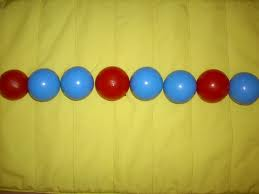Dividing ballsQuestion :

You have 100 balls (50 Red ,50 Blue). You have to divide them in 2 bags in any way you like. One of the 2 bags will be chosen at random and a ball will be chosen out of it at random.  How you divide them to maximize the probability of selecting a Red ball.

Bag 1  ( 1 Red, 0 Blue)

Bag 2 ( 49 Red, 50 Blue)

Hence the probability of selecting a Red will be

Prob(Bag 1)x Prob(Red under Bag 1)+ Prob(Bag 2)x Prob(Red under Bag 2)

(1/2) x (1) + (1/2)x(49/100)

0.5+0.245

0.745

Slightly less than 75%